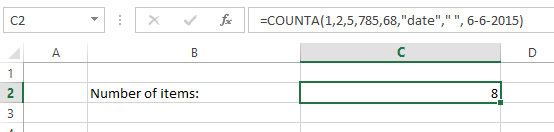# How to Use Counta Function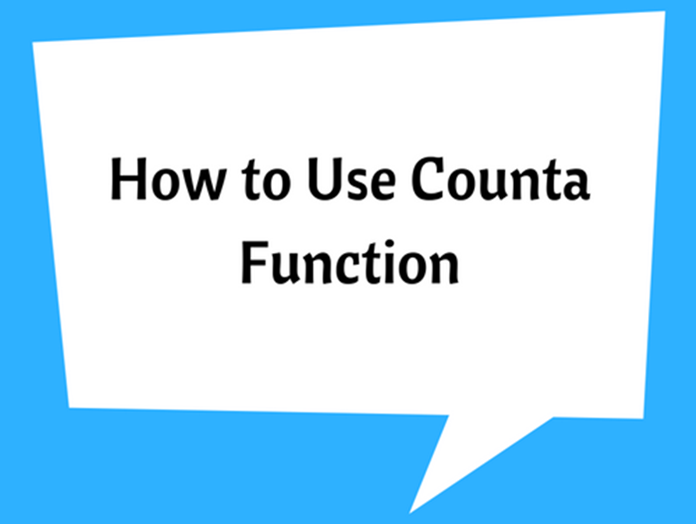The COUNTA function is usually used for counting the non-empty cells in a given cell range. It counts cells that contains any type of data including numerical data, text, dates, logic values, empty text “ ” and even error values such as “#DIV/0!”.

The syntax is a very simple one as explained below.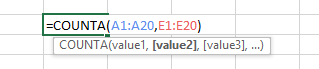value1: is the first cells range where you want to count non empty cells.

[value2]: is optional which represents the next cells range where you want to count non empty cells.

These arguments can be arranged up to a maximum of 255 values (i.e. value255).

Example: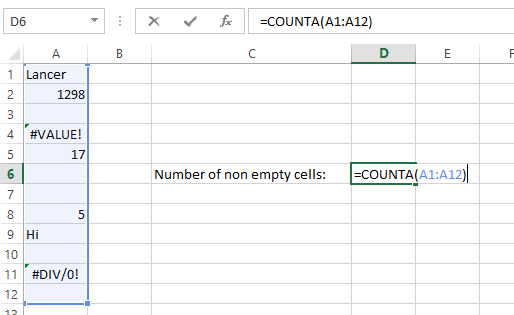Note that cell A7 is a non-empty cell as it contains an empty text “ “. So it is also counted as non-empty cell.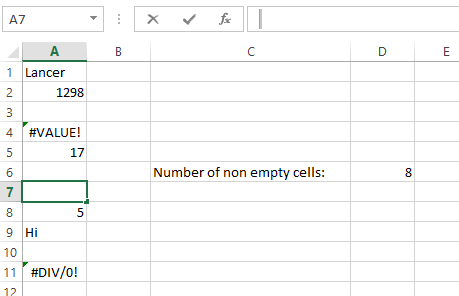If you don’t want to count cells with text, empty text, error cells or any data other than numbers then you can use COUNT() function. COUNT() only counts cells that contains numbers.

When you want to count only some cells that satisfy a certain criteria then use COUNTIF() of COUNTIFS() functions.

COUNT() function can be used to count items. See the following example. However, which is less useful.